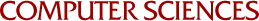## An Interactive Graphical System for the Approximation of Partial Differential Equations

Paul S. LaFata
1971

An interactive graphical system which can be used to approximate bivariate functions or to obtain approximate solutions to partial differential equations is described. The function or differential equation is entered symbolically, so the system may be used by someone with no programming experience. The basic mathematical technique is to assume the approximate solution is a linear combination of user selected functions, and to use linear programming to determine the coefficients of these functions. The approximate solution and error information can be graphically displayed in several different ways, and based on these results various parameters may be modified on line so as to obtain a better approximation.